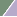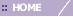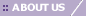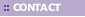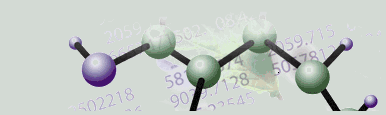# Spearman Rank Correlation

Overview

Spearman Rank Correlation measures the correlation between two sequences of values. The two sequences are ranked separately and the differences in rank are calculated at each position, i. The distance between sequences X = (X1, X2, etc.) and Y = (Y1, Y2, etc.) is computed using the following formula: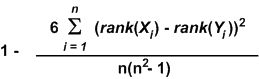Where Xi and Yi are the ith values of sequences X and Y respectively.

The range of Spearman Correlation is from -1 to 1. Spearman Correlation can detect certain linear and non-linear correlations. However, Pearson Correlation may be more appropriate for finding linear correlations.

Related Topics:

Clustering Overview